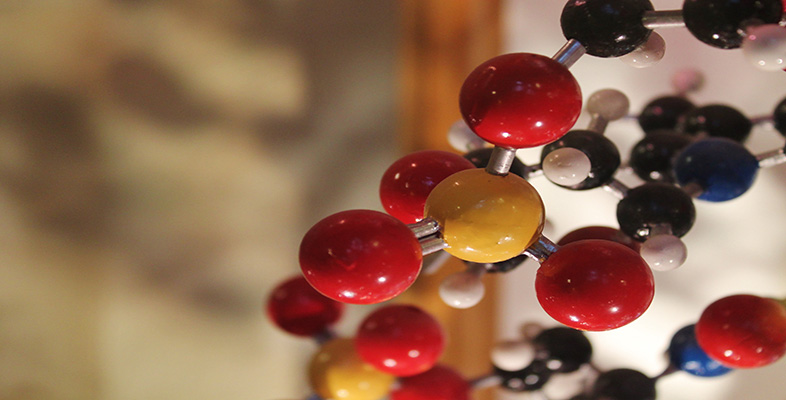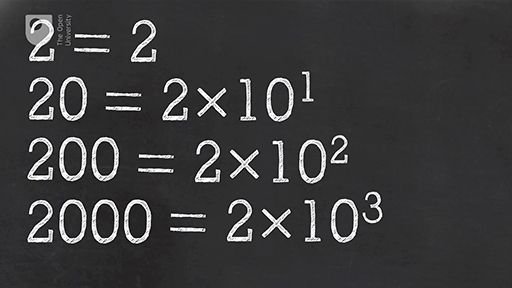Basic science: understanding numbers

Start this free course now. Just create an account and sign in. Enrol and complete the course for a free statement of participation or digital badge if available.

Free course

# 1.7 Scientific notationSkip transcript

#### Transcript

TEACHER
Big numbers like this are cumbersome and difficult to read. Just watch all those zeros going by. Lots of them, aren't there?
If scientists had to read or write them out in full when they were discussing things like the molecules of water in a swimming pool or the distance to the Orion nebula, it would take up pages of books. The same is true of very small numbers like this one. And it's an important number-- the charge on a single electron. But you could spend so much time counting the zeros you lose track of what the number was about.
So, how do scientists solve the problem of very big and very small numbers? In fact, scientists use a really simple device called scientific notation that allows them to abbreviate these numbers so that they're easy to write down and work with. The numbering system we use works in tens. That's the basis of our counting system.
So 2, 20, 200, and 2,000 are increasingly large numbers. They're also each 10 times larger than the previous number. You could think of that set of numbers as 2, 2 multiplied by 10, 2 multiplied by 100, and 2 multiplied by 1,000. But that doesn't help much for very large numbers.
The same numbers could also be written as 2, 2 times 10, 2 times 10 times 10, and 2 times 10 times 10 times 10. Think of that as 2, 2 times 10 one time, 2 times 10 two times, and 2 times 10 three times. Scientists write that with a superscript and describe it as 'to the power of'. This last number is, therefore, 2 times 10 to the power three. This is scientific notation.
You can write any number like this, and they're all roughly the same length, even 2 times 10 100 times. The basic form of scientific notation is a number. Let's call this number A multiplied by 10 to the power of another number. Let's call this number B. B tells you how many times 10 shall be multiplied by itself.
Let's start with the number 500. You can visualise the process of scientific notation by focusing on the decimal point of the number and imagining it hopping over digits until there's only one digit left in front of it. Also note that the number left in front of the decimal needs to be greater than 0 and 1 less than 10. This is an important point which we'll get to later.
So, for 500, the action number could also be written 5 times 10 times 10. Another way to think about that is that the decimal point is at the right hand end and it needs to hop over two digits until there's only one digit remaining in front of it. The number of hops is two, which is, therefore, the number B. So the number 500 is written as 5 times 10 to the power of 2 in scientific notation.
How about writing the number 7,500 in scientific notation? This time, the decimal point hops over three digits so the number B is a 3. If we remove the zeros that the decimal point hopped over, we're left with 7.5. So the number A is replaced by 7.5. The number, 7,500, can be written in scientific notation as 7.5 times 10 to the power of 3.
We can check that this is correct by working out the individual components. 10 times 10 times 10 equals 1,000. And 7.5 times 1,000 gives us our original number of 7,500.
Small numbers can be written in a very similar way. Let's start with the number 0.05. The only number here greater than 0 is the 5 at the right hand end of the number. This means that the decimal point needs to hop over digits to the right until the 5 is in front of it. To do this, the decimal point hops over two numbers. Again, the number B is replaced by a 2.
However, because we've moved towards the right, we're moving toward smaller numbers. And therefore, a minus sign is required before the 2.
If we remove all the zeros that we hopped over and the one that was before the decimal place, we're left with the number 5. This becomes our number A. So the number 0.05 can be written in scientific notation as 5 times 10 to the power of minus 2. Again, we can check that this is correct by working out the individual components. 10 to the power of minus 2 is actually 0.1 times 0.1 which equals 0.01. And 0.01 multiplied by 5 results in 0.05.
You should now be able to write our original very large number and very small number in scientific notation. For the large number, the decimal point hops over 22 digits and is left with a 1 in front of it. Don't worry, you won't have to do this very often. Most numbers like this are normally already written in scientific notation. In scientific notation, this number is 1 times 10 to the 22.
For the very small number, the decimal point needs to hop right over 19 digits and leaves us with 1.6. In scientific notation, this is written as 1.6 times 10 to the minus 19, which is the electric charge of a single electron. It's an important number that's much easy to remember in scientific notation.
End transcript

Interactive feature not available in single page view (see it in standard view).

## Activity 1.3 Trying out scientific notation

This video introduces scientific notation – the way scientists write very big and very small numbers. Try to think of large or small science-related things and practise writing them in scientific notation.

There’s an additional challenge in this activity … how do you write the superscripts? When you compose your post, you should see a button which looks like this: X2. To make a number superscript, highlight it using your mouse and then press this button.

Try it for yourself by posting a large number using scientific notation.